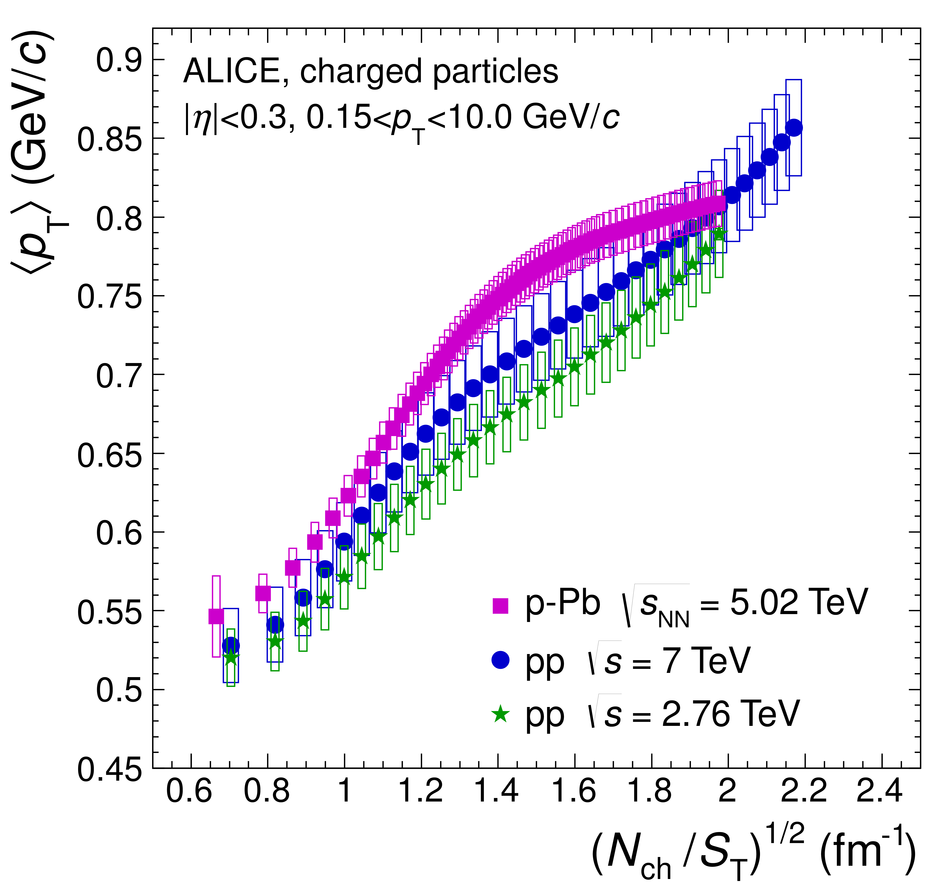# Figure 4

 Average transverse momentum $\mpt$ for $0< \pt< 10.0$ GeV/$c$ as a function of the scaled charged-particle multiplicity in p-Pb and pp collisions for $|\eta|< 0.3$. The boxes represent the systematic uncertainties on $\mpt$. The statistical errors are negligible.